# Equivalent Fractions Worksheet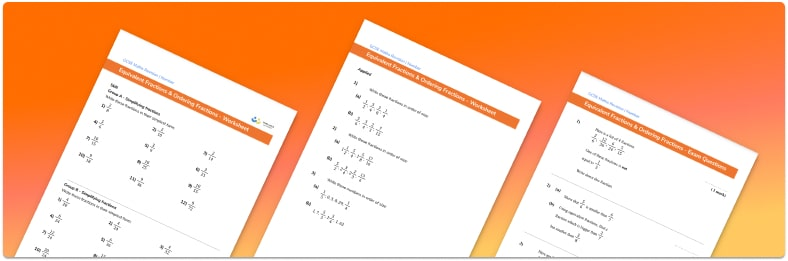• Section 1 of the equivalent fractions & ordering fractions worksheet contains 36 skills based equivalent fractions & ordering fractions questions, in 3 groups to support differentiation
• Section 2 contains 4 applied equivalent fractions & ordering fractions questions with a mix of worded problems and deeper problem solving questions
• Section 3 contains 4 foundation and higher level GCSE exam style equivalent fractions & ordering fractions questions
• Answers and a mark scheme for all equivalent fractions & ordering fractions questions
• Follows variation theory with plenty of opportunities for students to work independently at their own level
• All questions created by fully qualified expert secondary maths teachers
• Suitable for GCSE maths revision for AQA, OCR and Edexcel exam boards

• This field is for validation purposes and should be left unchanged.

You can unsubscribe at any time (each email we send will contain an easy way to unsubscribe). To find out more about how we use your data, see our privacy policy.

### Equivalent fractions at a glance

Equivalent fractions are fractions which have the same value despite having different numerators and denominators. For example, the fraction 1 over 2 is equivalent to the fraction 3 over 6. To find an equivalent fraction we need to multiply or divide the numerator and denominator by the same number.

A fraction is in its simplest form when there are no common factors that can be cancelled from the numerator and denominator. When simplifying fractions we need to divide the numerator and denominator by the same number, for example let’s look at the given fraction of 15 over 20. This can be simplified to 3 over 4 by dividing the top and bottom by the common factor 5. There are no other whole numbers that are factors of 3 and 4, so the fraction is in its simplest form.

When comparing fractions, we can use the concept of equivalent fractions to rewrite the fractions with a common denominator. For example, for two different fractions such as 3 over 5 and 2 over 7, we need to look at the denominators and find the lowest common multiple of both numbers. In this case the lowest common denominator of 5 and 7 is 35 and so we need to write both fractions with a denominator of 35 and then calculate the missing numerators.

Looking forward, students can then progress to additional number worksheets, for example aor a. There are also lots of additional fractions resources available.For more teaching and learning support on Number our GCSE maths lessons provide step by step support for all GCSE maths concepts.

## Related worksheets

Converting Mixed Numbers To Improper Fractions Worksheet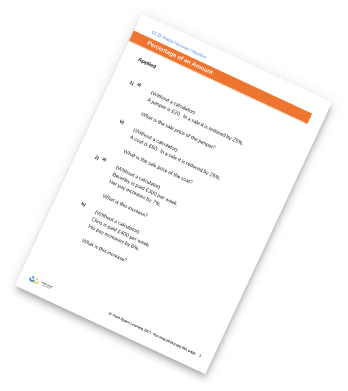Dividing Fractions Worksheet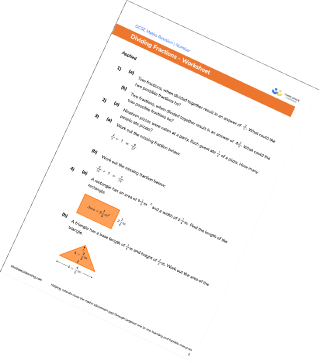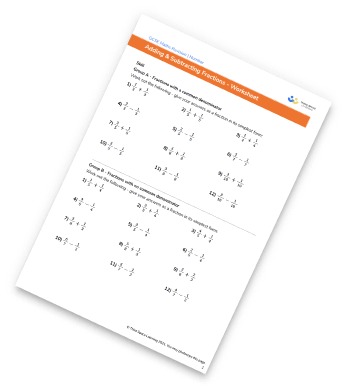Multiplying And Dividing Fractions Worksheets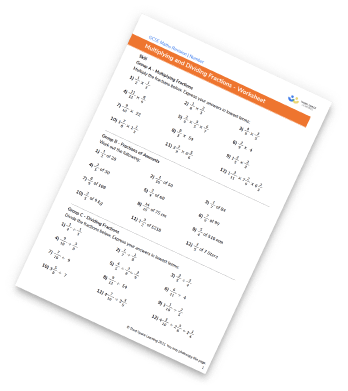## Do you have KS4 students who need more focused attention to succeed at GCSE?There will be students in your class who require individual attention to help them succeed in their maths GCSEs. In a class of 30, it’s not always easy to provide.

Help your students feel confident with exam-style questions and the strategies they’ll need to answer them correctly with our dedicated GCSE maths revision programme.

Lessons are selected to provide support where each student needs it most, and specially-trained GCSE maths tutors adapt the pitch and pace of each lesson. This ensures a personalised revision programme that raises grades and boosts confidence.

Find out more